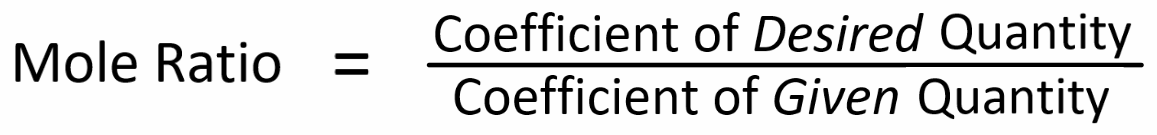# All Topics     |   Breslyn.org   |     Stoichiometry Topics

### The Mole Ratio and Balanced Equations

A major reason we balance equations is to find the ratio between substances in the equation. The coefficients represent the ratios of substances. We often think of the coefficients as moles. The ratio is called the Mole Ratio.

The mole ratio is the key to equation stoichiometry.

### Key Mole Ideas:

• The mole ratio is the only time we use the coefficients from a balanced equation in stoichiometry.

• The mole ratio is at the center of equation stoichiometry.

• In solving typical stoichiometry problems we convert grams of the given substance to moles, then use the mole ratio to get moles of the desired quantity, and then convert these moles to grams.

More Help and Practice:

### Thinking Tools

In stoichiometry, we only use the coefficients in the Mole Ratio. We can think of the coefficients as moles!One way to find the the mole ratio is:We get the coefficients from the balanced equation.

Go To Top
Subscribe